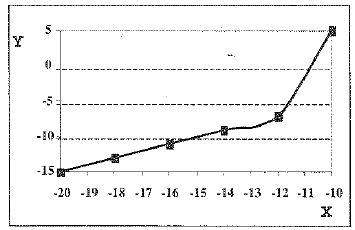Aptitude Test Math Samples
 Updated On: Aug 235, 2019

The Aptitude Test is a combination of basic Algebra and Reading. Below are some Algebra sample questions that you can use to help you study for the Aptitude Test.

Sample Algebra and Functions

1. Consider the following formula:

A = B + 3(4 - C)

If B equals 5 and C equals 2, what is the value of A?

A) 7

B) 11

C) 12

D) 16

2. Consider the following formula:

= 3(x + 5) (x - 2)

Which of the following formulas is equivalent to this one?

A) y = 3x2 + 9x -30

B) y = x2 + 3x - 10

C) y = 3x2 + 3x -10

D) y = 3x2 + 3x - 30

3. Consider the following pattern of numbers:

110, 112 , 107, 109, 104

What is the next number in the pattern?

A) 97

B) 99

C) 106

D) 109

4. Consider the following formula:

a = 1/2b - 4

Which of the following statements is  true for this formula?

A) When the value of b is less than 8, a is negative.

B) When the value of b is greater than 8, a is negative.

C) When the value of b is less than 8, a is positive.

D) When the value of b is greater than 4, a is positive.

5. Consider the following table:

 X Y 0 -5 1 -4 2 -3 3 -2 4 -1 5 0 6 1

Which of the following choices represents the same relationship as demonstrated in this table?

 X Y 10 -40 20 -30 30 -20 40 -10

B) Y = X/2 - 5

C) Y is equivalent to the difference between the value of X and a constant C, where C equals 5.

D)